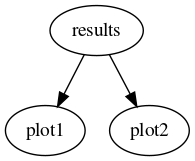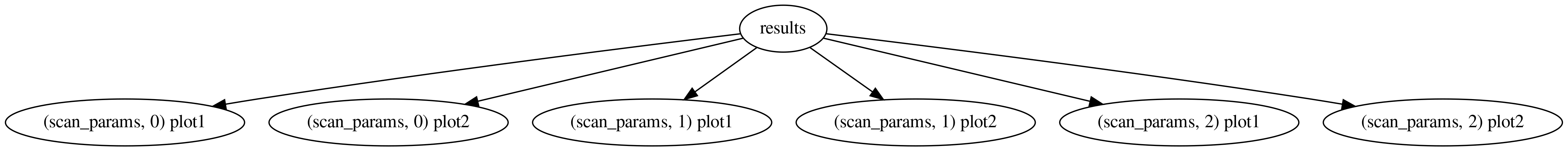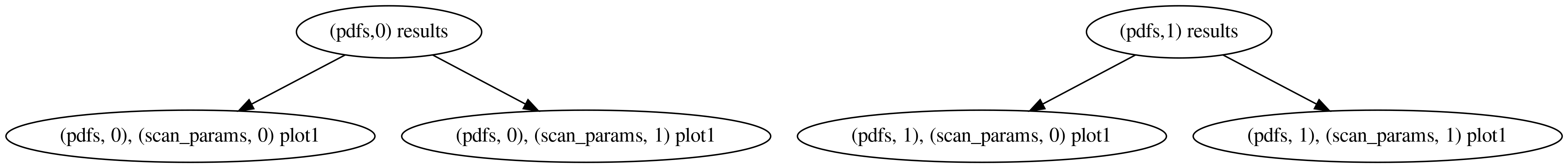# Resolving dependencies

Dependencies are resolved automatically by reportengine when the client applications follow a certain convention.

A few things that validphys needs to do are:

• Provide a declarative interface where the user specifies only the amount of information needed to specify the requirements.

• Be usable as a normal Python library.

• Reuse the computations that are common to several actions.

In order to do all that, one declares “provider modules” (which is done in validphys.app), which are nothing but normal Python files containing functions (and thus can be used as a library). The convention in reportengine is that a parameter with the same name as a provider function specifies that that function is a dependency.

Imagine we want to have two plotting tools plot1 and plot2, each of which takes as an argument the result of the same computation, results, which in turn need a PDF set entered by the user to be computed. One would declare the functions as follows:

```def results(pdf):
#Compute the results
...

def plot1(results):
#Take the result and produce a plot of type 1.
...

def plot2(results):
#Take the result and produce a plot of type 2.
...
```

Then, an input card like the following:

```pdf: NNPDF30_nlo_as_0118

actions_:
- plot1
- plot2
```

would result in the following directed acyclic graph (or DAG):The important point to note is that parameter names determine the dependencies by default.

To address the inflexibility that results from the way we choose to automatically assign dependencies, each action is assigned a unique namespace specification. This allows one to specify actions with several different parameters. Let’s make the example above more complicated:

```def results(pdf):
#Compute the results
...

def plot1(results, parameter):
#Take the result and produce a plot of type 1.
...

def plot2(results, parameter):
#Take the result and produce a plot of type 2.
...
```

We can request a parameter scan like this:

```pdf: NNPDF30_nlo_as_0118

scan_params:
- parameter: 5
- parameter: 10
- parameter: 20

actions_:
- scan_params plot1
- scan_params plot2
```

which would result in the following computation:We have requested the two plots to be computed once in each of the three namespaces spanned by scan_params. The actions are in general not computed in the requested namespace, but rather in the outermost one that satisfies all the dependencies (there is also a unique private stack frame per action not shown in the figures above). That’s why, in the graph above, results appears only once: since it doesn’t depend on the value of parameter (it doesn’t appear in its signature), it is computed in the root namespace, rather than once in each of the scan_params namespaces. If we instead had this:

```pdfs:
- NNPDF40_nnlo_as_01180
- CT14nlo

scan_params:
- parameter: 5
- parameter: 10

actions_:
- pdfs::scan_params plot1
```

The corresponding graph would be:since results does depend on the pdf.